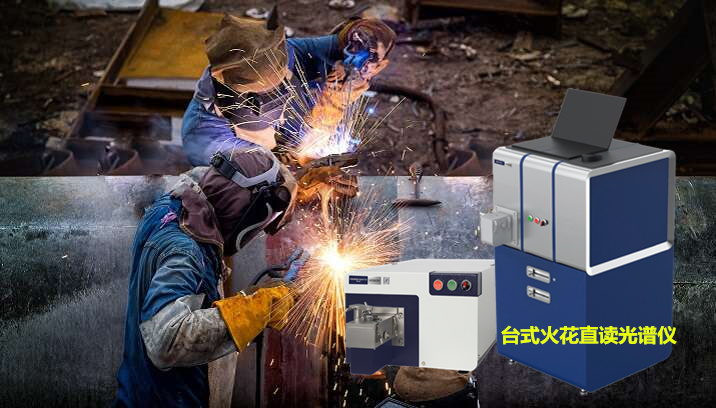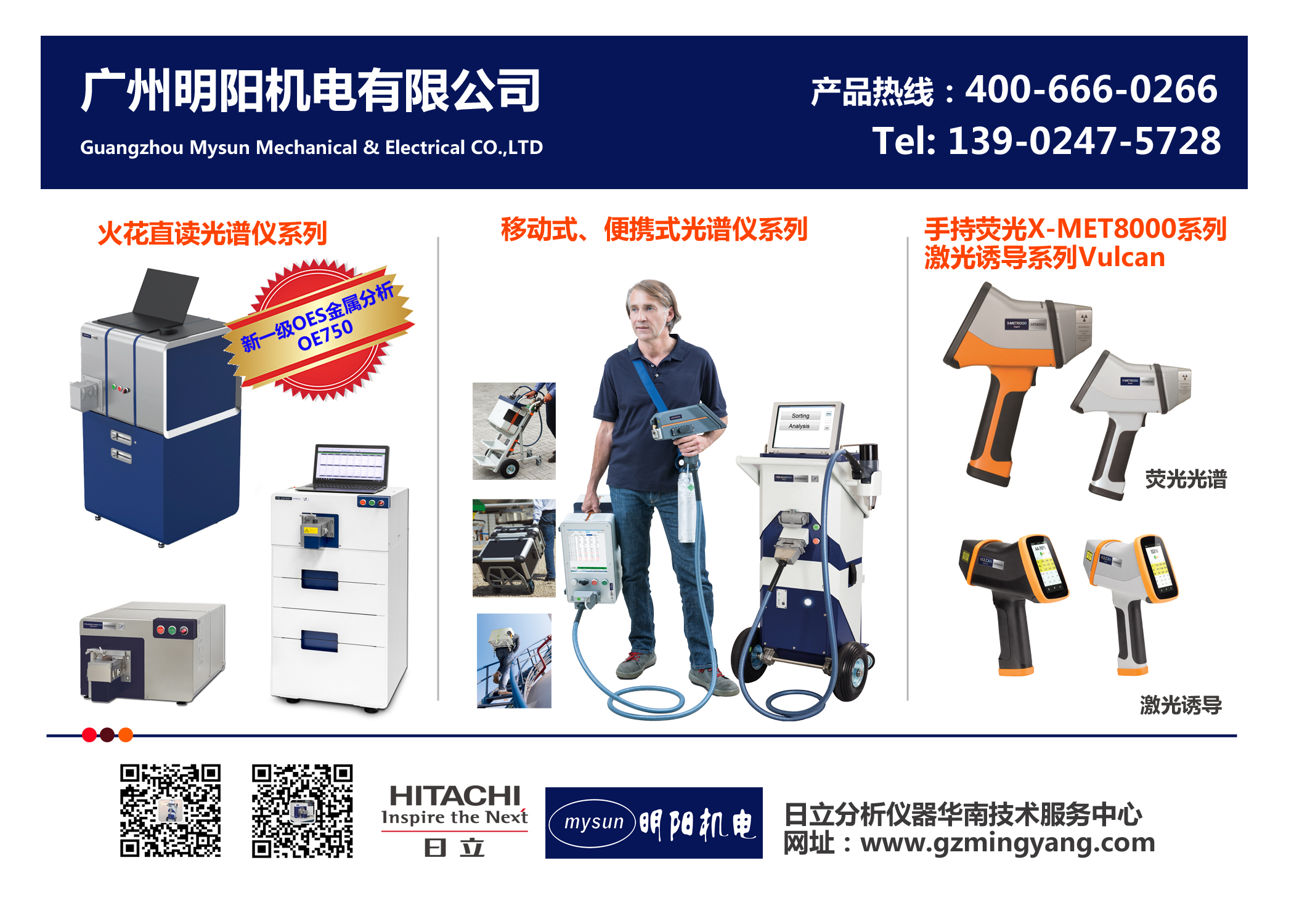碳当量（CE） 可焊性 最高达 0.35 极好 0.36–0.40 很好 0.41–0.45 良好 0.46–0.50 一般 0.36–0.40 很好 超过 0.50 不良

PCM = (%C)+((%Si)/30)+(((%Cr)+(%Cu)+(%Cr))/20)+((%Ni)/60)+((%Mo)/15)+((%V)/10)+((%B)*5)

Düren CEM:

CEM = (%C)+((%Si)/25)+(((%Mn)+(%Cu))/20)+(((%Cr)+(%V))/10)+((%Mo)/15) +((%Ni)/40)

Thyssen CET:

CET = (%C)+(((%Mn)+(%Mo))/10)+(((%Cr)+(%Cu))/20)+((%Ni)/40)

EN 10025-1 CEV:

CEV = (%C)+((%Mn)/6)+(((%Cu)+(%Ni))/15)+(((%Cr)+(%Mo)+(%V))/5)>